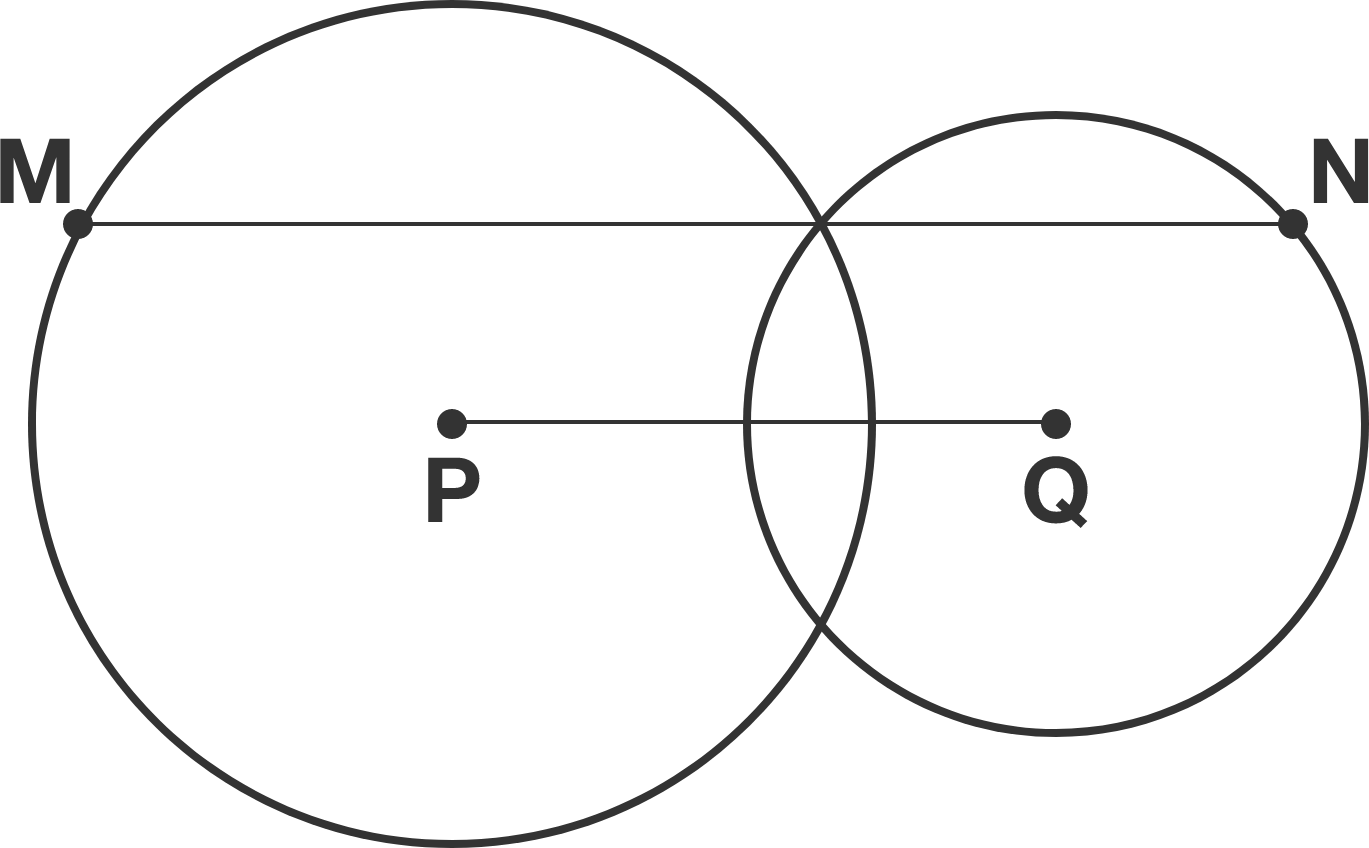# Blow of sight

Geometry Level 1

Two circles with respective centers $P$ and $Q$ intersect with each other, as shown below. Line segment $MN$ is parallel to $PQ$ and goes through one of the intersection points.

If $PQ=3,$ what is $MN?$×